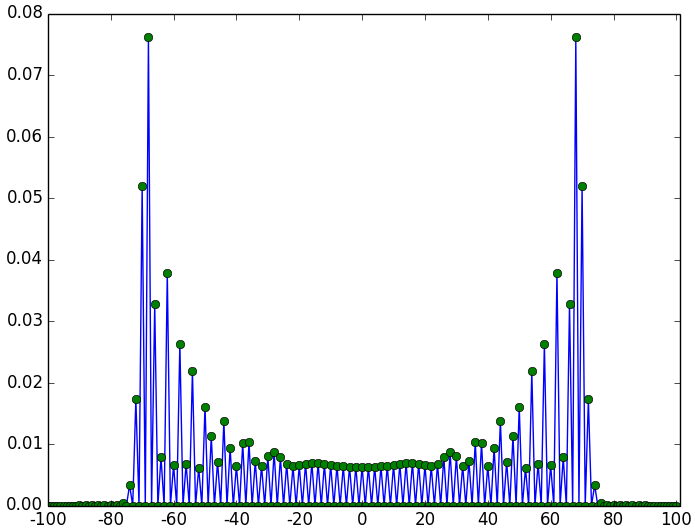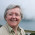## Saturday, 1 February 2014

### quantum walking

It’s possible to build a quantum random walk simulator in Python/NumPy with code that is very close to the mathematical definitions.  Here’s how.

First, we need to import NumPy (to do the array operations) and matplotlib (to visualise the results).
from numpy import *
from matplotlib.pyplot import *
We define the number of steps, $N$, that we are going to walk.  We also define the total number of different positions the walker can be in after $N$ steps.
N = 100      # number of random steps
P = 2*N+1    # number of positions

### a quantum coin

We toss a quantum coin to decide whether to go left or right (or a superposition).  In ket notation, we can write a general quantum coin as an arbitrary superposition of two states:
$$| coin \rangle = a | 0 \rangle_c + b | 1 \rangle_c; \mbox{ where} |a|^2 + |b|^2 = 1$$
The ket notation is a convenient shorthand for the actual vectors representing the state:
$$| 0 \rangle_c = \left( \begin{array}{c} 1 \\ 0 \end{array} \right);\ | 1 \rangle_c = \left( \begin{array}{c} 0 \\ 1 \end{array} \right)$$
So we can use a NumPy array to define a coin state:
coin0 = array([1, 0])  # |0>
coin1 = array([0, 1])  # |1>

We will see terms like $| 0 \rangle_c \langle 0 |$ in what follows.  This is the outer product of the relevant vectors, resulting in a matrix.  We can use NumPy to calculate these:
C00 = outer(coin0, coin0)  # |0><0|
C01 = outer(coin0, coin1)  # |0><1|
C10 = outer(coin1, coin0)  # |1><0|
C11 = outer(coin1, coin1)  # |1><1|

Quantum operators are unitary matrices.  The coin operator, that can be used to flip a quantum coin into a superposition, is:
$$\hat{C} = \frac{1}{\sqrt{2}} \left( | 0 \rangle_c \langle 0 | + | 0 \rangle_c \langle 1 | + | 1 \rangle_c \langle 0 | - | 1 \rangle_c \langle 1 | \right)$$
C_hat = (C00 + C01 + C10 - C11)/sqrt(2.)

### position on a line

In ket notation, we can write the fully general position of the walker on the line as an arbitrary superposition of the $P$ possible states:
$$| posn \rangle = \sum_k \alpha_k | k \rangle_p ; \mbox{ where} \sum_k | \alpha_k |^2 = 1$$

### shift (step) operator

The step operator moves left or right along the line, depending on the value of the coin:
$$\hat{S} = | 0 \rangle_c \langle 0 | \otimes \sum_k | k+1 \rangle_p \langle k | + | 1 \rangle_c \langle 1 | \otimes \sum_k | k-1 \rangle_p \langle k |$$
We assume the line is actually on a circle, so the positions at the ends wrap around.  However, we will always make the circle big enough so that this doesn’t happen during a walk.  The tensor product $\otimes$ is implemented with the NumPy kron operation:
ShiftPlus = roll(eye(P), 1, axis=0)
ShiftMinus = roll(eye(P), -1, axis=0)
S_hat = kron(ShiftPlus, C00) + kron(ShiftMinus, C11)

### walk operator

The walk operator combines the coin operator on the coin state, and a step operator on the combined coin and position state:
$$\hat{U} = \hat{S} \left( \hat{C} \otimes \hat{\bf I}_p \right)$$
U = S_hat.dot(kron(eye(P), C_hat))

### initial state

Let’s take the initial state of the system to be a coin in a superposition of left and right, and the walker at position 0:
$$| \psi \rangle_0 = | coin \rangle_0 \otimes | posn \rangle_0 = \frac{1}{\sqrt{2}} \left( | 0 \rangle_c + i | 1 \rangle_c\right) \otimes | 0 \rangle_p$$
posn0 = zeros(P)
posn0[N] = 1     # array indexing starts from 0, so index N is the central posn
psi0 = kron(posn0,(coin0+coin1*1j)/sqrt(2.))

### state after $N$ steps

Then walking $N$ steps is just applying the walk operator $N$ times:
$$| \psi \rangle_N = \hat{U}^N | \psi \rangle_0$$
psiN = linalg.matrix_power(U, N).dot(psi0)
And we’re done! $| \psi \rangle_N$ is the state of the system after $N$ random quantum steps.

### measurement operator

We can measure the state at position $k$ using the measurement operator
$$\hat{M}_k = \hat{\bf I}_c \otimes | k \rangle_p \langle k |$$
We can use this to build up an array of probabilities, by taking the modulus squared of the state value at each position. (We can calculate the whole distribution in one go in simulation, but we would only get one measurement per experiment on the real quantum system.)
prob = empty(P)
for k in range(P):
posn = zeros(P)
posn[k] = 1
M_hat_k = kron( outer(posn,posn), eye(2))
proj = M_hat_k.dot(psiN)
prob[k] = proj.dot(proj.conjugate()).real

### plot the distribution

We can then plot the probabilities.
fig = figure()

plot(arange(P), prob)
plot(arange(P), prob, 'o')
loc = range (0, P, P / 10) #Location of ticks
xticks(loc)
xlim(0, P)
ax.set_xticklabels(range (-N, N+1, P / 10))

show()

For $N=100$ we getprobability distribution for a quantum random walk with $N=100$, symmetric initial coin

The maximum probability occurs at $\approx N/\sqrt{2}$.

If instead of starting with a symmetric initial coin, we start with $|0\rangle_c$, we getprobability distribution for a quantum random walk with $N=100$, initial coin $|0\rangle$
In neither case do these look anything like the bell curve of a classical random walk, with its maximum probability at $0$.  If you are drunk and trying to stagger home, best be quantum!

What I find most impressive about the Python is how closely we can make the code follow the mathematical formalism thoughout.

1.Thanks for this...saves me writing it myself for my Computing 2 students, I'll just direct therm here instead! -- Viv

2.Thanks a lot for this.

3.Could someone help me understand the ShiftPlus and ShiftMinus operators written in terms of roll function?

4.Both Pyhton as well as the quantum walk problem are new to me. Could someone help me to understand the ShiftPlus and ShiftMinus operators written in terms of some mysterious roll function?

1."roll" is a numpy function, with definition and examples defined here:
https://docs.scipy.org/doc/numpy-1.13.0/reference/generated/numpy.roll.html

5.Hi Susan,
Thank you very much for sharing this great work.
I have question please, If I want to use the same shift but add to it the mod

6.quick fyi, the code returns a float P unless we use int, as bellow :-)
loc = range (0, P, P // 10) #Location of ticks
ax.set_xticklabels(range (-N, N+1, P // 10))

1.Ah yes -- the code is old enough to be in python2!